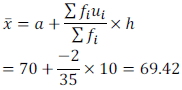CBSE Board NCERT Solutions for Class 10th Mathematics Chapter14 : Statistics
CBSE NCERT Solutions for Class Ten Mathematics Chapter14 – Statistics
CBSE NCERT Solutions For Class 10th Maths Chapter 14

NCERT Solutions for Class X Maths Chapter 14 Statistics – Mathematics CBSE
Exercise 14.1

1. A survey was conducted by a group of students as a part of their environment awareness programme, in which they collected the following data regarding the number of plants in 20 houses in a locality. Find the mean number of plants per house.

Which method did you use for finding the mean, and why?
Solution:

Mean can be calculated as follows: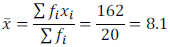In this case, the values of fi and xi are small hence direct method has been used.

2. Consider the following distribution of daily wages of 50 workers of a factory.

Find the mean daily wages of the workers of the factory by using an appropriate method.

Solution: In this case, value of xi is quite large and hence we should select the assumed mean method.

Let us take assumed mean a = 150

Now, mean of deviations can be calculated as follows: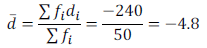Mean can be calculated as follows:

x = d + a = -4.8 + 150 = 145.20

3. The following distribution shows the daily pocket allowance of children of a locality. The mean pocket allowance is Rs. 18. Find the missing frequency f.

Solution:

We have;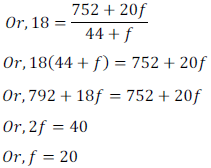4. Thirty women were examined in a hospital by a doctor and the number of heart beats per minute were recorded and summarized as follows. Find the mean heart beats per minute for these women, choosing a suitable method.

Solution:

Now, mean can be calculated as follows: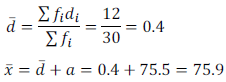5. In a retail market, fruit vendors were selling mangoes kept in packing boxes. These boxes contained varying number of mangoes. The following was the distribution of mangoes according to the number of boxes.

Find the mean number of mangoes kept in a packing box. Which method of finding the mean did you choose?

Solution:

Mean can be calculated as follows: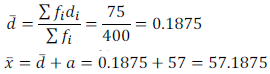In this case, there are wide variations in fi and hence assumed mean method is used.

6. The table below shows the daily expenditure on food of 25 households in a locality.

Find the mean daily expenditure on food by a suitable method.

Solution:

Mean can be calculated as follows: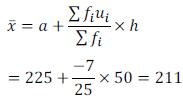7. To find out the concentration of SO2 in the air (in parts per million, i.e. ppm), the data was collected for 30 localities in a certain city and is presented below:

Find the mean concentration of SO2 in the air.
Solution:

Mean can be calculated as follows: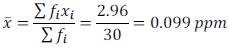8. A class teacher has the following absentee record of 40 students of a class for the whole term. Find the mean number of days a student was absent.

Solution:

Mean can be calculated as follows: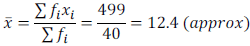9. The following table gives the literacy rate (in percentage) of 35 cities. Find the mean literacy rate.

Solution:

Mean can be calculated as follows: